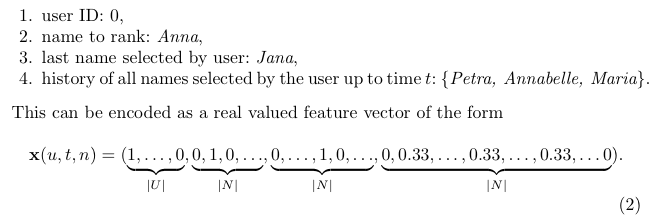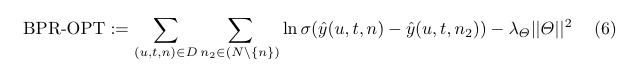## A Bayesian Personalised Ranking Example: Factor Models for Recommending Given Names

Immanuel Bayer and Steffen Rendle

ECML PKDD Discovery Challenge 2013 (offline track).

This paper provides an interesting example of using factorisation machines for implicit feedback via the Bayesian personalised ranking (BPR) optimisation criterion.

The challenge was to recommend first names (e.g. to soon-to-be parents). Participants were provided with the browsing history of users on the name selection website Nameling. So the items to be recommended are names.

Users are considered together with their browsing history, consisting of the name they looked at last and the list of all the names they looked at up to the time $t$ in question. The combination of a user (in this complete sense) at time $t$ and a candidate name is vectorised as follows:The (order 2) factorisation machine assigns a score to the combination as follows:where $p$ is the rank of this vectorisation, $w_0 \in \mathbb{R}$, $w \in \mathbb{R}^p$ and $V \in \mathbb{R}^{p \times k}$ are model parameters to be learned. These parameters are learned via stochastic gradient ascent of the following pair-wise learning objective:where $D$ is the set of (user u, time t, name n) tuples where u has browsed n before time t, $N$ is the set of all possible names and $\sigma$ is the sigmoid function. Only a single name is chosen from $N \setminus \{ n \}$ for each update. These negative samples are drawn according to their estimated rank (this part is quite difficult to do efficiently).

In the above, we have purposefully omitted the “prefix smoothing” step, since we are mainly interested in the a simple factorisation machine example. The details are in the paper.

Remarks
This recommender did very well in the challenge. However, I don’t find the examples given in the paper very impressive (though I have not seen the examples given by others). My suspicion is that the FM approach is very strong, but that there is no good way to make first name recommendations using the provided dataset. A more effective dataset would be e.g. first name x product interaction on a large e-commerce site. This would do a much better job of capturing the social meaning of names, but could go out of date very quickly.

## Logistic Matrix Factorization for Implicit Feedback Data

Christopher Johnson, Spotify, 2014

PDF

A new matrix factorisation model for behavioural recommendation in the case of implicit feedback is presented.

User-item interactions are encoded in a non-negative interaction matrix. The question as to whether a user-item interaction occurred is then treated as a problem of binary classification. User-item pairs for which an interaction has occurred are regarded as positive outcomes with confidence in constant proportion to the value in the interaction matrix, while the absence of an interaction is regarded as a negative outcome. This binary classification is task is then leveraged to train user- and item-vectors. These vectors reside in dually paired spaces. The dot-product of the vectors, combined with user- and item- bias terms, is then fed into the sigmoid function. What we are really doing is looking for a low-rank approximation to a bilinear form via the sigmoid function.

Confidence values (proportional to the values in the interaction matrix) are used as powers of their corresponding factor in the maximum likelihood function. The constant proportion that defines the confidence values from the interaction matrix is a tuning parameter, but is typically chosen so that the positive outcomes balance the negative outcomes in total confidence. Thus the likelihood function is weighed according to the entries of the interaction matrix. The weighted likelihood function is then maximised using alternating gradient ascent. This optimisation is batch. Negative sampling can be used to speed up convergence, and the confidence parameter is decreased proportionally.

Evalutation
They use a fractional rank type metric to evaluate performance at each iteration. For each user, the interaction probability is computed for each item, and the rank of the target item in this list is determined. This is then averaged over a set-aside collection of user-item pairs. Given that batch gradient descent is used, this is not prohibitively expensive.

The author reports that this logistic matrix factorisation model performs better in low rank than the implicit MF model of Koren et al 2008, though both give a similar fractional rank in high ranks.

Implementation
A very basic implementation in Python is available. The implementation uses AdaGrad to dynamically chose a step size at each iteration, just as is described in the paper. The paper mentions a Spark or Hadoop based implementation, but I couldn’t find this published anywhere.